Win up to 100% scholarship on Aakash BYJU'S JEE/NEET courses with ABNAT Win up to 100% scholarship on Aakash BYJU'S JEE/NEET courses with ABNAT

# Raoult’s Law

Raoult’s law has been named after François-Marie Raoult, a French chemist, who while conducting an experiment, found out that when substances were mixed in a solution, the vapour pressure of the solution decreased simultaneously. Raoult’s law was established in the year 1887 and is also considered as the law of thermodynamics.

We will further take an in-depth look at Raoult’s law and understand the principle behind the law as well as its application and limitations in this lesson.

## What Is Raoult’s Law?

Raoult’s law states that a solvent’s partial vapour pressure in a solution (or mixture) is equal or identical to the vapour pressure of the pure solvent multiplied by its mole fraction in the solution.

Mathematically, Raoult’s law equation is written as:

Psolution = ΧsolventP0solvent

Where,

Psolution = vapour pressure of the solution
Χsolvent  = mole fraction of the solvent
P0solvent = vapour pressure of the pure solvent

Further, we will understand the principle behind the law by looking at the example below.

Consider a solution of volatile liquids A and B in a container. Because A and B are both volatile, there would be both particles of A and B in the vapour phase.

Hence, the vapour particles of both A and B exert partial pressure, which contributes to the total pressure above the solution.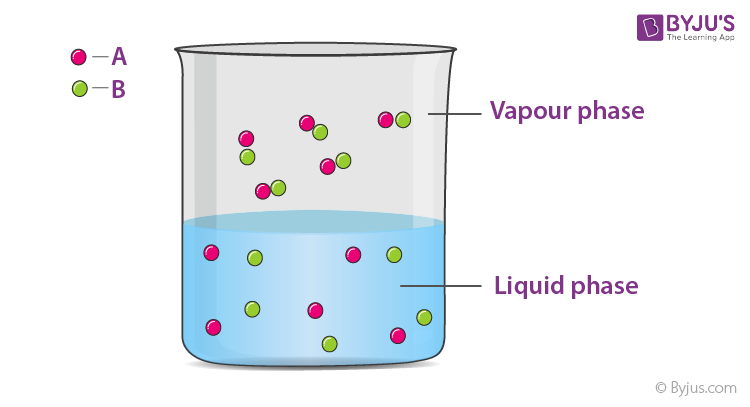Raoult’s law further states that at equilibrium,

$$\begin{array}{l}{{P}_{A}}=P_{A}^{{}^\circ }{{x}_{A}},{{P}_{B}}=P_{B}^{{}^\circ }{{x}_{B}}\end{array}$$

Where PA is the partial pressure of A.

P°A is the vapour pressure of pure A at that temperature.

xA is the mole fraction of A in the liquid phase.

Similarly, PB, P°B, xB

Hence,

$$\begin{array}{l}{{P}_{T}}={{P}_{A}}+{{P}_{B}}\left( Dalton’s\,Law \right)\end{array}$$
$$\begin{array}{l}=P_{A}^{{}^\circ }{{x}_{A}}+P_{B}^{{}^\circ }{{x}_{B}}\end{array}$$
$$\begin{array}{l}=P_{A}^{{}^\circ }+{{x}_{B}}\left( P_{B}^{{}^\circ }-P_{A}^{{}^\circ } \right)\end{array}$$

## What Is the Importance of Raoult’s Law?

Assume that we have a closed container filled with a volatile liquid A. After some time, due to evaporation, vapour particles of A will start to form. Then as time passes, the vapour particles of A will be in dynamic equilibrium with the liquid particles (on the surface). The pressure exerted by the vapour particles of A at any particular temperature is called the vapour pressure of A at that temperature.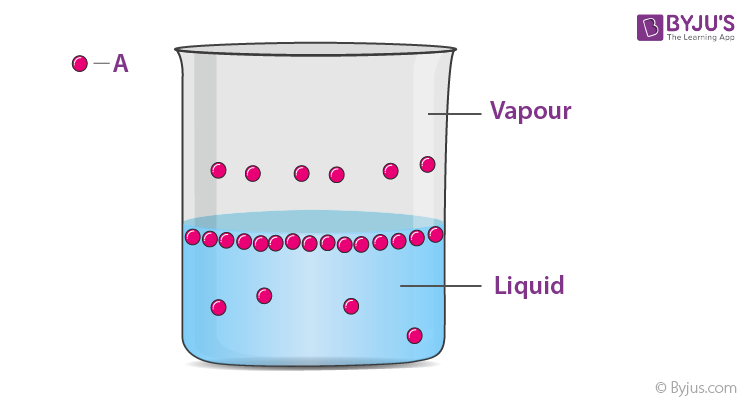Vapour pressure is exhibited by all solids and liquids and depends only on the type of liquid and temperature.

Now, imagine that we are adding another liquid, B (solute), to this container. This will result in B particles occupying the space between A particles on the surface of the solution.

For any given liquid, there are a fraction of molecules on the surface which will have sufficient energy to escape to the vapour phase.

Now, since we have a lesser number of A particles on the surface, the number of vapour particles of A in the vapour phase will be lesser. This will result in lower vapour pressure of A.

Now, if we assume that B is volatile as well, we will have a lesser number of B particles in the vapour phase as compared to pure liquid B.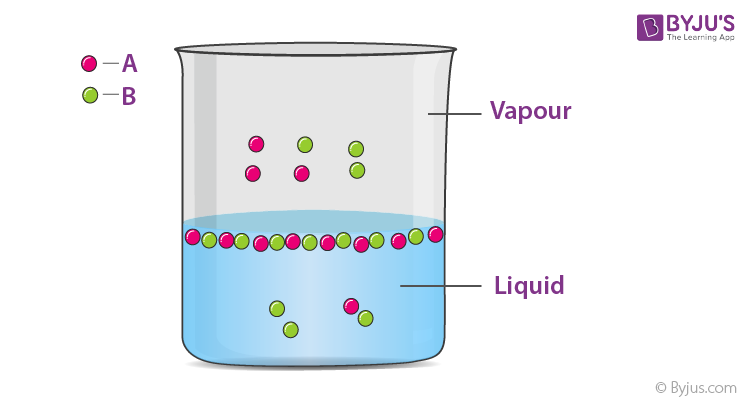This new pressure (partial pressure) of each (A and B) is given by Raoult’s law and depends on the concentration of each component in the liquid phase.

$$\begin{array}{l}{{P}_{A}}\propto {{X}_{A}},\,\,\,{{P}_{B}}\propto {{X}_{B}}\end{array}$$
$$\begin{array}{l}={{X}_{A}}P_{A}^{o}\,\,\,\,\,={{X}_{B}}P_{B}^{o}\end{array}$$

From Raoult’s law, it is evident that as the mole fraction of a component reduces, its partial pressure also reduces in the vapour phase.

The graphs below show that pressure is the mole fraction for A and B.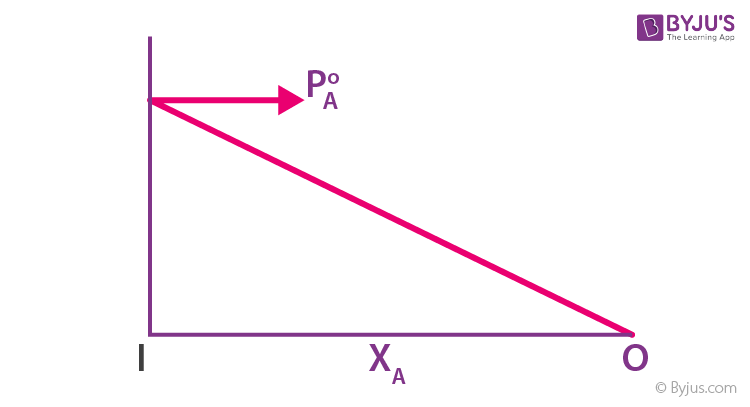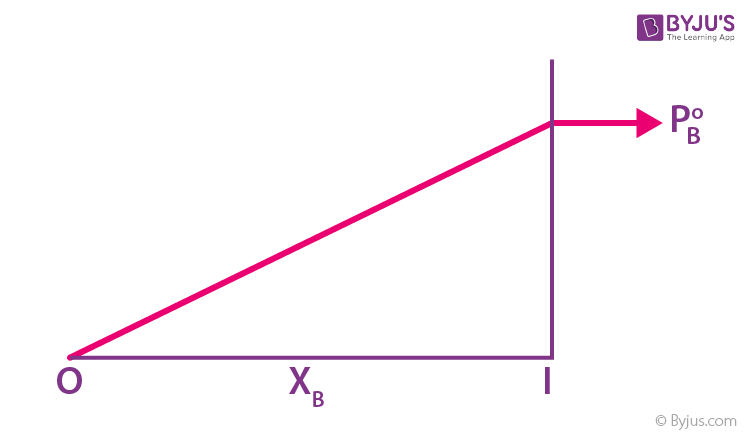Combining both graphs,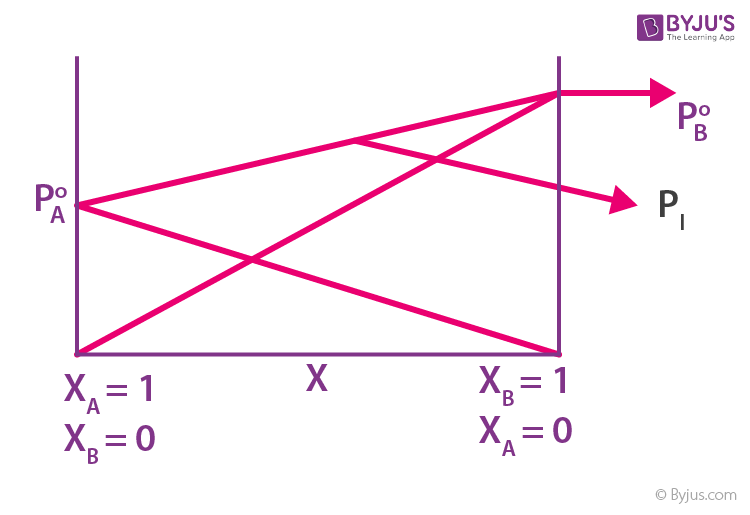In the above diagram, we have also added the graph for the total vapour pressure of the solution, i.e. PA + PB.

As far as the application of the law goes, it is also useful in calculating the molecular mass of an unknown solute.

## Raoult’s Law and Its Relationship with Other Laws

Raoult’s law is also quite similar to the ideal gas law. The only exception of Raoult’s law is that it applies to solutions. If you have read about the ideal gas law, you know that it assumes the ideal behaviour of gases in which the intermolecular forces that are present between dissimilar molecules are zero or non-existent. On the other hand, Raoult’s law assumes that the intermolecular forces that exist between different molecules and similar molecules are equal.

Raoult’s law can also be applied to non-ideal solutions. However, this is done by incorporating several factors where we have to consider the interactions between molecules of different substances.

If we take a perfectly ideal system consisting of an ideal liquid and ideal vapour, we can further derive a very useful equation by combining Raoult’s law and Dalton’s law.

$$\begin{array}{l}x_{i}=\frac{yi Ptotal}{P_{i}^{*}}\end{array}$$

Here,

xi = mole fraction of component i in the solution,

yi = mole fraction in the gas phase.

What we can deduce from this equation is that when we have an ideal solution with pure components, each of them will have a different vapour pressure. Furthermore, in the gas phase, the component will have a higher pure vapour pressure, while the solution will have a lower pure vapour pressure.

## Limitations of Raoult’s Law

There are a few limitations to Raoult’s law.

• Raoult’s law is apt for describing ideal solutions. However, ideal solutions are hard to find, and they are rare. Different chemical components have to be chemically identical equally.
• Since many of the liquids that are in the mixture do not have the same uniformity in terms of attractive forces, these types of solutions tend to deviate away from the law.

There is either a negative or a positive deviation. The negative deviation occurs when the vapour pressure is lower than expected from Raoult’s law. An example of negative deviation is a mixture of chloroform and acetone or a solution of water and hydrochloric acid.

Alternatively, positive deviation takes place when the cohesion between similar molecules is greater or when it exceeds adhesion between unlike or dissimilar molecules. Both components of the mixture can easily escape from the solution. An example of positive deviation includes the mixtures of benzene and methanol or ethanol and chloroform.

### FAQs

1. Is Raoult’s law valid for all types of solutions?

Solution:

No, Raoult’s law is valid only in the case of ideal solutions.

In an ideal solution, the solvent-solute interaction is the same as a solvent–solvent or solute–solute interaction. This implies both the solute and the solvent takes the same amount of energy to escape to the vapour phase as when they are in their pure states.

2. Is the graph of pressure vs mole fraction always a straight line?

Solution:

If the solution is ideal, the 3 graphs (PA, PB and PT) will always be a straight line because

$$\begin{array}{l}{{P}_{A}}={{X}_{A}}P_{A}^{o}\end{array}$$

$$\begin{array}{l}{{P}_{B}}={{X}_{B}}P_{B}^{o}\end{array}$$

$$\begin{array}{l}{{P}_{T}}=P_{e}^{o}{{x}_{e}}+P_{m}^{o}{{x}_{m}}.\end{array}$$

P°e is the vapour pressure of pure ethanol.

P°m is the vapour pressure of pure methanol.

xe and xm are mole fractions of ethanol and methanol.

No. of moles of ethanol (ne)

$$\begin{array}{l}=\frac{60}{46}=1.3\,mol\end{array}$$

No. of moles of methanol (nm)

$$\begin{array}{l}=\frac{40}{32}=1.25\,mol\end{array}$$

$$\begin{array}{l}{{x}_{e}}=\frac{{{n}_{e}}}{{{n}_{e}}+{{n}_{m}}}=\frac{1.33}{\left( 1.33+1.25 \right)}=0.51\end{array}$$

$$\begin{array}{l}{{x}_{m}}=\frac{{{n}_{B}}}{{{n}_{e}}+{{n}_{m}}}=\frac{1.25}{\left( 1.33+1.25 \right)}=0.49\end{array}$$

Therefore,

$$\begin{array}{l}\,\,{{P}_{T}}=\left( 0.51\times 44.5 \right)+\left( 0.49\times 88.7 \right)=66.2\,mmHg\end{array}$$

## Video Lesson – Vapour Pressure and Raoult’s LawTest Your Knowledge On Raoults Law!